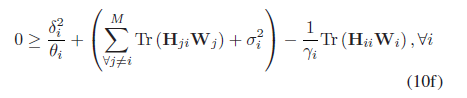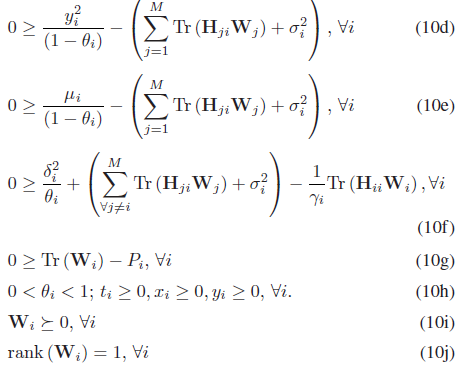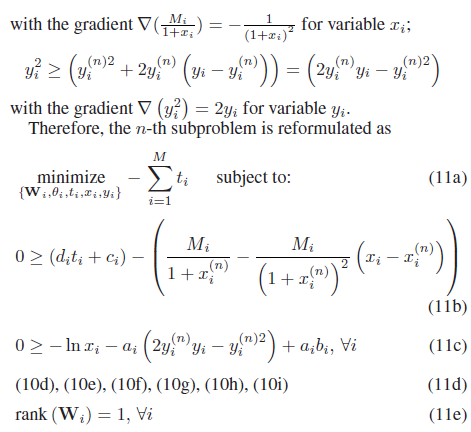# Im writing a code for energy harvesting but the results is disappointing in one constraint

``````    (quad_over_lin(zeta,theta(i))+sum((real(TRC2)+segma))-(inv_pos(sinr(k))*(real(trace(H(:,:,i,i)*H(:,:,i,i)'*W(:,:,i))))))<=0;
``````

hence .
TRC2 = TRC2 + trace(H(:,:,j,i)*H(:,:,j,i)’*W(:,:,j));What specifically is the issue? Are you saying that the constraint is not satisfied? Solvers only have to satisfyconstrains to within a tolerance. For instance, if the LHS of the constraint winds up being 1e-9, that would be considered to satisfy the constraint. If you are not satisfied by this, you can add a small positive number, such as 1e-6, to the LHS in order to ensure that your original constraint is not violated at all.

Also, do the solver and CVX report that the problem is solved to optimality?

Also, if any of zeta, theta, TRC2,sinrr, H, W are CVX expressions (and not CVX variables or MATLAB variables), when you evaluate the constraint after CVX concludes, it may appear that the constraint is not satisfied even if it i. That is because CVX expressions are not guaranteed to have their optimal value, but instead must be recomputed starting from CVX variables. However, the expressions are evaluated correctly during the optimization.

If none of these resolve your difficulty, please post the solver and CVX output, as well as the evidence that the results is “disappointing in one constraint”.

1 Like

thank you very much for rapid reply and for your concern and valuable informations
the specifically is the issue is
Efficient Energy Transmission on a SWIPT Interference Channel Under
Non-linear energy harvesting Models
i changed cvx version to 2.2, Build 1148 (62bfcca) and i got no error and the results :

## Cones | Errors | Mov/Act | Centering Exp cone Poly cone | Status --------±--------------------------------±-------- 0/ 0 | 0.000e+00 0.000e+00 0.000e+00 | Infeasible

Status: Unbounded
Optimal value (cvx_optval): +Inf

the cvx part of the code is:

cvx_begin
variables t(1,M) x(1,M) y(1,M) %slack variables.
variable W(N,N,M) complex semidefinite % beamforming or precoding vector.
variable theta(1,M) %power splitting factor 0<theta<1 .

`````` maximize (sum(t))
subject to

for i = 1:M
0<=theta(i);
theta(i)<=1;
x(i)>=0;
y(i)>=0;
t(i)>=0;
((di*t(i))+ci)-((mi/(1+x_old(i)))-(mi/square((1+x_old(i))))*(x(i)-x_old(i)))<=0;
(-log(x(i)))-(ai*(2*y_old(i)*y(i)-square(y_old(i))))+(ai*bi)<=0;
(W(:,:,i)) == hermitian_semidefinite(N);
real(trace(W(:,:,i)))-p<=0;
TRC = cvx(0);
TRC2 = cvx(0);
for j = 1:M
TRC = TRC + trace(H(:,:,j,i)*H(:,:,j,i)'*W(:,:,j));
if i~=j
TRC2 = TRC2 + trace(H(:,:,j,i)*H(:,:,j,i)'*W(:,:,j));
end

end
for k=1:lk
th=(1-theta(i));
(mu*inv_pos(th))-sum((real(TRC)+segma))<=0;
end
end
cvx_end
x_old = x;
y_old = y;

MHE(1:5) = sum(t);     % the maximum harvested energy.
``````

![image|488x64](upload://tUEN8YrtytbDBuqOuQBvosomS0n.png)The solver is solving the dual problem, and reports it is infeasible, so CVX conclude that your original (primal) problem is unbounded. See https://yalmip.github.io/debuggingunbounded/ for tips on diagnosing that.

Given that your model involves log of CVX variable, I recommend that you either use Mosek 9.2 as solver, if that is available to you, oi if not, follow the advice in CVXQUAD: How to use CVXQUAD's Pade Approximant instead of CVX's unreliable Successive Approximation for GP mode, log, exp, entr, rel_entr, kl_div, log_det, det_rootn, exponential cone. CVXQUAD's Quantum (Matrix) Entropy & Matrix Log related functions

1 Like

best wishes for you

sir i tried mosek as a solver but the results as follow:

CVX Warning:
Models involving “log” or other functions in the log, exp, and entropy
family are solved using an experimental successive approximation method.
This method is slower and less reliable than the method CVX employs for
other models. Please see the section of the user’s guide entitled
The successive approximation method
for more details about the approach, and for instructions on how to
suppress this warning message in the future.

## Calling Mosek 9.1.9: 162 variables, 69 equality constraints For improved efficiency, Mosek is solving the dual problem.

MOSEK Version 9.1.9 (Build date: 2019-11-21 11:34:40)
Platform: Windows/64-X86

## MOSEK warning 62: The A matrix contains a large value of -1.1e+10 in constraint ‘’ (37) at variable ‘’ (8). MOSEK warning 62: The A matrix contains a large value of -1.9e+10 in constraint ‘’ (38) at variable ‘’ (8). MOSEK warning 62: The A matrix contains a large value of -1.9e+10 in constraint ‘’ (42) at variable ‘’ (8). MOSEK warning 62: The A matrix contains a large value of 1.8e+10 in constraint ‘’ (43) at variable ‘’ (8). MOSEK warning 62: The A matrix contains a large value of 2.5e+12 in constraint ‘’ (45) at variable ‘’ (8). MOSEK warning 62: The A matrix contains a large value of 1.5e+10 in constraint ‘’ (46) at variable ‘’ (8). MOSEK warning 62: The A matrix contains a large value of 1.4e+16 in constraint ‘’ (47) at variable ‘’ (8). MOSEK warning 62: The A matrix contains a large value of 2.3e+12 in constraint ‘’ (42) at variable ‘’ (28). MOSEK warning 62: The A matrix contains a large value of 1.1e+12 in constraint ‘’ (44) at variable ‘’ (28). MOSEK warning 62: The A matrix contains a large value of 2.5e+16 in constraint ‘’ (45) at variable ‘’ (28). Mosek error: MSK_RES_ERR_HUGE_AIJ (A numerically huge value is specified for an element in A.)

Status: Error
Optimal value (cvx_optval): NaN

Reference to non-existent field ‘sol’.

Error in cvx_mosek

Error in cvxprob/solve (line 429)
[ x, status, tprec, iters, y ] = shim.solve( At, b, c, cones, quiet, prec, solv.settings, eargs{:} );

Error in cvx_end (line 88)
solve( prob );

Error in EHEMANS (line 100)
cvx_end

The scaling in your problem is terrible. You need to change units so that non-zero numbers in model coefficients are closer to 1 in magnitude. Mosek (nor any other solver) doesn’t like very large or small magnitude numbers. Fortunately, Mosek warns you about this.

If you for instance have

exp(20*(x+y))

then do not write

exp(20)*exp(x+y)

I know these mathematically is the same, but the first one does not involve insane constants.

@Erling How huge does a number have to be for Mosek to issue

## Mosek error: MSK_RES_ERR_HUGE_AIJ (A numerically huge value is specified for an element in A.)

as opposed to a warning, such as

## MOSEK warning 62: The A matrix contains a large value of 2.5e+16 in constraint ‘’ (45) at variable ‘’ (28).

@Mark_L_Stone You can decide!But 1e+20 by default

https://docs.mosek.com/9.2/capi/parameters.html#mosek.dparam.data_tol_aij_huge

@Michal_Adamaszek Thanks. I can tell you that out in the real world, there are many people* who would fix such an error, including on systems involving life or death of large numbers of people, by setting `MSK_DPAR_DATA_TOL_AIJ_HUGE` to `inf`. Voilà, problem solved, ha ha. (well, it would be funny if large numbers of people might not die as a result).

I have a question, what should be used instead of “task” in `MSK_putdouparam(task, MSK_DPAR_DATA_TOL_AIJ_HUGE, 1.0e20)`
sorry，this is my first use of mosek.